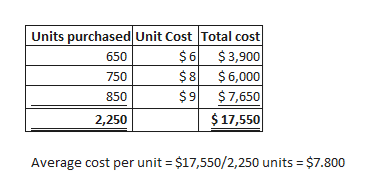# In its first month of operations, Skysong, Inc. made three purchases of merchandise in the following sequence: (1) 650 units at \$6, (2) 750 units at \$8, and (3) 850 units at \$9.  Calculate average unit cost. (Round answer to 3 decimal places, e.g. 5.125.)Average unit cost \$enter an average unit cost in dollars   Compute the cost of the ending inventory under the average-cost method, assuming there are 550 units on hand at the end of the period. (Round answer to 0 decimal places, e.g. 125.)The cost of the ending inventory \$enter the cost of the ending inventory in dollars

Question
10 views
In its first month of operations, Skysong, Inc. made three purchases of merchandise in the following sequence: (1) 650 units at \$6, (2) 750 units at \$8, and (3) 850 units at \$9.

Calculate average unit cost. (Round answer to 3 decimal places, e.g. 5.125.)

 Average unit cost \$enter an average unit cost in dollars

Compute the cost of the ending inventory under the average-cost method, assuming there are 550 units on hand at the end of the period. (Round answer to 0 decimal places, e.g. 125.)

 The cost of the ending inventory \$enter the cost of the ending inventory in dollars
check_circle

Step 1

Determine average co...help_outlineImage TranscriptioncloseUnits purchased Unit Cost Total cost \$6 \$3,900 \$6,000 \$8 \$7,650 \$9 \$17,550 650 750 850 2,250 Average cost per unit = \$17,550/2,250 units \$7.800 CO fullscreen

### Want to see the full answer?

See Solution

#### Want to see this answer and more?

Solutions are written by subject experts who are available 24/7. Questions are typically answered within 1 hour.*

See Solution
*Response times may vary by subject and question.
Tagged in